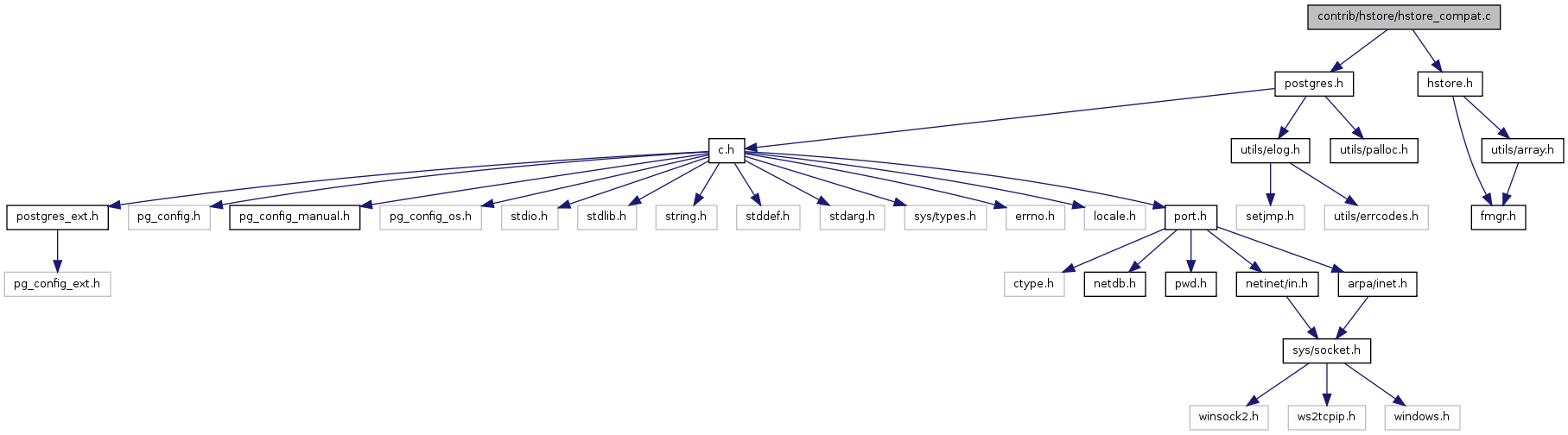# hstore_compat.c File Reference

`#include "postgres.h"`
`#include "hstore.h"`
Include dependency graph for hstore_compat.c:Go to the source code of this file.

## Data Structures

struct  HOldEntry

## Functions

static int hstoreValidNewFormat (HStore *hs)
static int hstoreValidOldFormat (HStore *hs)
PG_FUNCTION_INFO_V1 (hstore_version_diag)
Datum hstore_version_diag (PG_FUNCTION_ARGS)

## Function Documentation

 Datum hstore_version_diag ( PG_FUNCTION_ARGS )

Definition at line 362 of file hstore_compat.c.

```{
HStore     *hs = (HStore *) PG_DETOAST_DATUM(PG_GETARG_DATUM(0));
int         valid_new = hstoreValidNewFormat(hs);
int         valid_old = hstoreValidOldFormat(hs);

PG_RETURN_INT32(valid_old * 10 + valid_new);
}
```

 HStore* hstoreUpgrade ( Datum orig )

Definition at line 236 of file hstore_compat.c.

```{
HStore     *hs = (HStore *) PG_DETOAST_DATUM(orig);
int         valid_new;
int         valid_old;
bool        writable;

/* Return immediately if no conversion needed */
if ((hs->size_ & HS_FLAG_NEWVERSION) ||
hs->size_ == 0 ||
(VARSIZE(hs) < 32768 && HSE_ISFIRST((ARRPTR(hs)))))
return hs;

valid_new = hstoreValidNewFormat(hs);
valid_old = hstoreValidOldFormat(hs);
/* Do we have a writable copy? */
writable = ((void *) hs != (void *) DatumGetPointer(orig));

if (!valid_old || hs->size_ == 0)
{
if (valid_new)
{
/*
* force the "new version" flag and the correct varlena length,
* but only if we have a writable copy already (which we almost
* always will, since short new-format values won't come through
* here)
*/
if (writable)
{
HS_SETCOUNT(hs, HS_COUNT(hs));
HS_FIXSIZE(hs, HS_COUNT(hs));
}
return hs;
}
else
{
elog(ERROR, "invalid hstore value found");
}
}

/*
* this is the tricky edge case. It is only possible in some quite extreme
* cases (the hstore must have had a lot of wasted padding space at the
* end). But the only way a "new" hstore value could get here is if we're
* upgrading in place from a pre-release version of hstore-new (NOT
* contrib/hstore), so we work off the following assumptions: 1. If you're
* moving from old contrib/hstore to hstore-new, you're required to fix up
* any potential conflicts first, e.g. by running ALTER TABLE ... USING
* col::text::hstore; on all hstore columns before upgrading. 2. If you're
* moving from old contrib/hstore to new contrib/hstore, then "new" values
* are impossible here 3. If you're moving from pre-release hstore-new to
* hstore-new, then "old" values are impossible here 4. If you're moving
* from pre-release hstore-new to new contrib/hstore, you're not doing so
* as an in-place upgrade, so there is no issue So the upshot of all this
* is that we can treat all the edge cases as "new" if we're being built
* as hstore-new, and "old" if we're being built as contrib/hstore.
*
* XXX the WARNING can probably be downgraded to DEBUG1 once this has been
* beta-tested. But for now, it would be very useful to know if anyone can
* actually reach this case in a non-contrived setting.
*/

if (valid_new)
{
#if HSTORE_IS_HSTORE_NEW
elog(WARNING, "ambiguous hstore value resolved as hstore-new");

/*
* force the "new version" flag and the correct varlena length, but
* only if we have a writable copy already (which we almost always
* will, since short new-format values won't come through here)
*/
if (writable)
{
HS_SETCOUNT(hs, HS_COUNT(hs));
HS_FIXSIZE(hs, HS_COUNT(hs));
}
return hs;
#else
elog(WARNING, "ambiguous hstore value resolved as hstore-old");
#endif
}

/*
* must have an old-style value. Overwrite it in place as a new-style one,
* making sure we have a writable copy first.
*/

if (!writable)
hs = (HStore *) PG_DETOAST_DATUM_COPY(orig);

{
int         count = hs->size_;
HEntry     *new_entries = ARRPTR(hs);
HOldEntry  *old_entries = (HOldEntry *) ARRPTR(hs);
int         i;

for (i = 0; i < count; ++i)
{
uint32      pos = old_entries[i].pos;
uint32      keylen = old_entries[i].keylen;
uint32      vallen = old_entries[i].vallen;
bool        isnull = old_entries[i].valisnull;

if (isnull)
vallen = 0;

new_entries[2 * i].entry = (pos + keylen) & HENTRY_POSMASK;
new_entries[2 * i + 1].entry = (((pos + keylen + vallen) & HENTRY_POSMASK)
| ((isnull) ? HENTRY_ISNULL : 0));
}

if (count)
new_entries.entry |= HENTRY_ISFIRST;
HS_SETCOUNT(hs, count);
HS_FIXSIZE(hs, count);
}

return hs;
}
```

 static int hstoreValidNewFormat ( HStore * hs ) ` [static]`

Definition at line 119 of file hstore_compat.c.

```{
int         count = HS_COUNT(hs);
HEntry     *entries = ARRPTR(hs);
int         buflen = (count) ? HSE_ENDPOS(entries[2 * (count) - 1]) : 0;
int         vsize = CALCDATASIZE(count, buflen);
int         i;

if (hs->size_ & HS_FLAG_NEWVERSION)
return 2;

if (count == 0)
return 2;

if (!HSE_ISFIRST(entries))
return 0;

if (vsize > VARSIZE(hs))
return 0;

/* entry position must be nondecreasing */

for (i = 1; i < 2 * count; ++i)
{
if (HSE_ISFIRST(entries[i])
|| (HSE_ENDPOS(entries[i]) < HSE_ENDPOS(entries[i - 1])))
return 0;
}

/* key length must be nondecreasing and keys must not be null */

for (i = 1; i < count; ++i)
{
if (HS_KEYLEN(entries, i) < HS_KEYLEN(entries, i - 1))
return 0;
if (HSE_ISNULL(entries[2 * i]))
return 0;
}

if (vsize != VARSIZE(hs))
return 1;

return 2;
}
```

 static int hstoreValidOldFormat ( HStore * hs ) ` [static]`

Definition at line 171 of file hstore_compat.c.

```{
int         count = hs->size_;
HOldEntry  *entries = (HOldEntry *) ARRPTR(hs);
int         vsize;
int         lastpos = 0;
int         i;

if (hs->size_ & HS_FLAG_NEWVERSION)
return 0;

/* New format uses an HEntry for key and another for value */
StaticAssertStmt(sizeof(HOldEntry) == 2 * sizeof(HEntry),
"old hstore format is not upward-compatible");

if (count == 0)
return 2;

if (count > 0xFFFFFFF)
return 0;

if (CALCDATASIZE(count, 0) > VARSIZE(hs))
return 0;

if (entries.pos != 0)
return 0;

/* key length must be nondecreasing */

for (i = 1; i < count; ++i)
{
if (entries[i].keylen < entries[i - 1].keylen)
return 0;
}

/*
* entry position must be strictly increasing, except for the first entry
* (which can be ""=>"" and thus zero-length); and all entries must be
* properly contiguous
*/

for (i = 0; i < count; ++i)
{
if (entries[i].pos != lastpos)
return 0;
lastpos += (entries[i].keylen
+ ((entries[i].valisnull) ? 0 : entries[i].vallen));
}

vsize = CALCDATASIZE(count, lastpos);

if (vsize > VARSIZE(hs))
return 0;

if (vsize != VARSIZE(hs))
return 1;

return 2;
}
```

 PG_FUNCTION_INFO_V1 ( hstore_version_diag )